## Example Questions

← Previous 1 3

### Example Question #1 : How To Find The Part From The Whole With Percentage

Your friend has 100 pounds of bacon and offers to share 45% of it with you.  If you promised your mom 30% and your cousin 25% of your share, how many pounds of bacon do you end up with?

13.5 pounds

11.25 pounds

20.25 pounds

24.75 pounds

45 pounds

20.25 pounds

Explanation:

Your share = 45% of 100 pounds of bacon = .45 * 100 = 45 pounds

For mom and cousin = 30% + 25% =  55%

Percent left for you = 100% - 55% = 45%

45% of 45 pounds = .45 * 45 = 20.25 pounds

### Example Question #2 : How To Find The Part From The Whole With Percentage

A container has ten liters of a twenty percent sucrose solution by volume. How many liters of the solution should be removed from the container and then replaced by the same volume of a forty percent sucrose solution, so that the final solution is ten liters of a twenty-five percent sucrose solution?

0.5

4

2.5

1.25

2

2.5

Explanation:

The final solution is going to have a concentration of twenty-five percent and a volume of 10 liters. The amount of sucrose in the solution is equal to the concentration (expressed as a decimal) multiplied by the total volume. Therefore, the final solution will have 25% • 10 = 0.25 • 10 = 2.5 liters of sucrose.

Let x be the amount of the solution that is removed and then replaced by the forty percent solution. The amount of twenty percent solution that will remain will be equal to 10 – x, because the final solution will still have ten liters. (The amount removed is replaced with the same amount, so the final volume of the solution is the same.)

So we have to get 2.5 liters of sucrose from the twenty percent solution and the forty percent solution combined. The amount of sucrose we will get from the twenty percent solution equals the volume of the twenty percent solution (which we established will be 10 – x) multiplied by twenty percent as a decimal.

Amount of sucrose from 20% solution = 0.20 • (10 – x)

The amount of sucrose we get from the forty percent solution will equal the product of x and forty percent (since we defined x as the amount of forty percent solution).

Amount of sucrose from 40% solution = 0.40x

Now, we need to add the amount of sucrose from the 20% and the 40% solutions, and then set this equal to 2.5, because ultimately we will have 2.5 liters of sucrose in the solution. We can write the equation as follows and then solve for x:

0.20 • (10 – x) + 0.40x = 2.5

Here is a table that we can also use to summarize the information discussed above.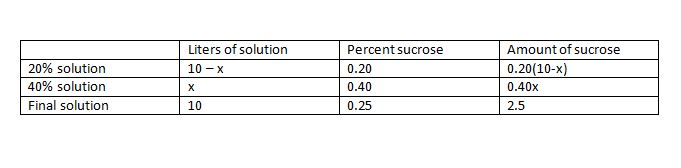0.20 • (10 – x) + 0.40x = 2.5

Distribute.

2 – 0.2x + 0.4x = 2.5

Combine x terms.

2 + 0.2x = 2.5

Subtract 2 from both sides.

0.2x = 0.5

Divide both sides by 0.2.

x = 2.5 liters

### Example Question #3 : How To Find The Part From The Whole With Percentage

A total of 200,000 votes were cast for two opposing candidates. If the losing candidate received 40% of the vote, how many votes did the winning candidate receive?

120,000

100,000

80,000

50,000

120,000

Explanation:

60% of 200,000 is 120,000

0.6 * 200,000 = 120,000

### Example Question #4 : How To Find The Part From The Whole With Percentage

Becky and Jason are running for class president, and each of the 30 students in the class voted. Becky received 60% of the votes. How many students voted for Jason?

12

15

21

18

12

Explanation:

If Becky received 60% of the vote, Jason must have received 40% of the vote. 40% is equal to 0.40. By multiplying the number of votes times the percentage, we can calculate how many votes Jason received.

0.40 * 30 = 12.

### Example Question #5 : How To Find The Part From The Whole With Percentage

I ate 30% of an entire pizza. My friend Sally ate 20% of the remaining pizza. What percentage of the original pizza is left?

56%

44%

50%

70%

14%

56%

Explanation:

After I eat 30% of the pizza, 70%, or 0.7, is left. Sally eats 20%, or 0.2 of that. So to compute how much of the original pizza Sally has eaten, we must multiply 0.7 by 0.2. We will arrive with 0.14 or 14%. Thus we subtract my the 30% of the pizza I ate and the 14% of the pizza Sally ate from 100% or the original whole, and we will arrive with 56%.

### Example Question #6 : How To Find The Part From The Whole With Percentage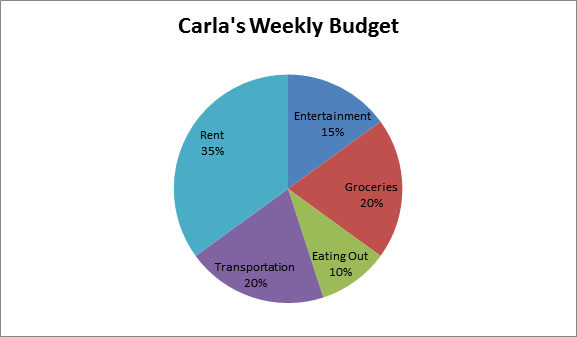The pie chart illustrates how Carla allocates her money each week.

If she spends $200 on groceries each week, how much does she spend on rent? Possible Answers: 450 750 1000 500 350 Correct answer: 350 Explanation: 1. 20% of Carla's whole budget is equal to$200. With this information, one can find Carla's weekly budget.

Set up the equation: 0.2b = 200 (b = budget).

b = 200/0.2 = 1000 = Carla's weekly budget

2. To find the amount Carla spends for rent, one needs to find what 35% of $1000 is. 0.35 x 1000 = 350 3. Because Carla spends 35% of her total budget on rent, she spends$350 on rent.

### Example Question #7 : How To Find The Part From The Whole With Percentage

There arepounds of cargo currently on a ship. Afterpounds have been taken from the ship and transferred to a warehouse, then, in terms ofand, what percent of cargo is still on the ship?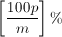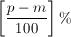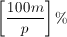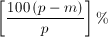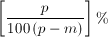Explanation:

If p is the number of pounds of cargo that is initially on the ship and m is the number of pounds of cargo that we transfer (or remove from the ship), we can find how many pounds of cargo are still on the ship after the transfer by the expression p – m. In order to model this as a percent, we would have to form a fraction, using the initial pounds of cargo, p, as the whole amount and p – m as the fractional part.

So,

we would get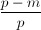as our fraction.

However, this problem asks us to find the percent, so we would simply multiply by 100will get our answer into percent form.

### Example Question #8 : How To Find The Part From The Whole With Percentage

Three salesmen, Gor, Levon, and Raffi, competed to sell the highest number of cars in the month of August. A total of 250 cars were sold.

Gor sold 100 cars. Levon sold 62% of the remaining cars, and Raffi sold the rest.

How many cars did Raffi sell?

39

75

93

57

77

57

Explanation:

We first subtract the 100 cars that Gor sold from the total of 250 sold. We are left with 150 cars, and we know that Levon sold 62% of them. 100% – 62% = 38%. Hence, Raffi sold 38% of 150 cars. 150 * 0.38 = 57

### Example Question #1071 : Sat Mathematics

A school is made up of 400 students. Each student must belong to one of the four clubs shown in the chart below. How many students are in chorus?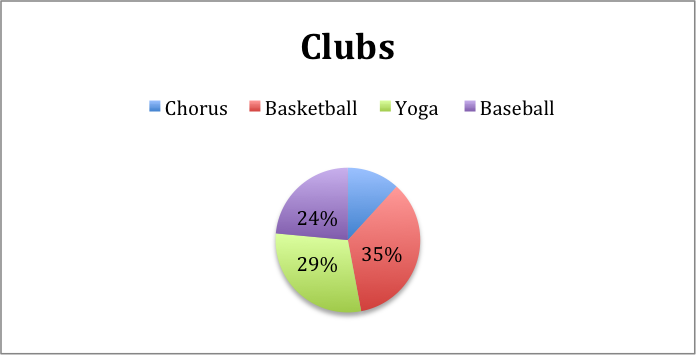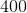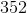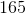Explanation:

You must first determine the percentage of the students in chorus. To do this, subtract the other percentages from 100: 100 – (35 + 29 + 24) = 12.

Therefore, 12% of the 400 students at this school attend chorus.

To determine the number of students in the chorus, multiply .12 (12%) by 400.

0.12(400) = 48

### Example Question #2 : How To Find The Part From The Whole With Percentage

What is 16% of 32?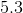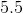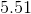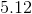Explanation:

There are two ways to solve this problem.

First, we can convert the given percentage to a decimal and multiple by the whole.

16% = 0.16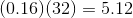Secondly, we could set up a proportion. We are given the whole from which a percentage is taken, so we can say: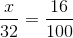To solve, cross multiply and simplify.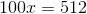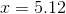← Previous 1 3